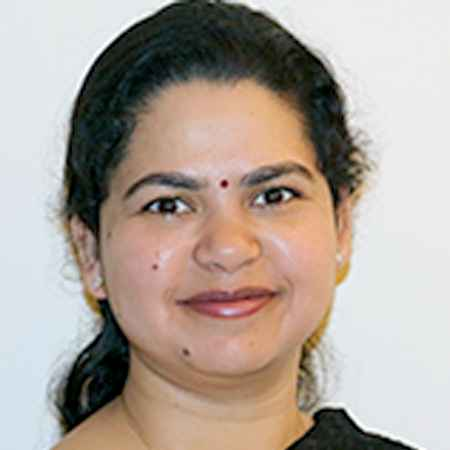X
Denoising and segmentation of 3D brain images
A. Noore
Published in
2009
Volume: 2

Pages: 561 - 567
Abstract
This paper presents an algorithm for medical 3D image denoising and segmentation using redundant discrete wavelet transform. First, we present a two stage denoising algorithm using the image fusion concept. The algorithm starts with globally denoising the brain images (3D volume) using Perona Malik's algorithm and RDWT based algorithms followed by combining the outputs using entropy based fusion approach. Next, a region segmentation algorithm is proposed using texture information and k-means clustering. The proposed algorithms are evaluated using brain 3D image/volume data. The results suggest that the proposed algorithms provide improved performance compared to existing algorithms.
••